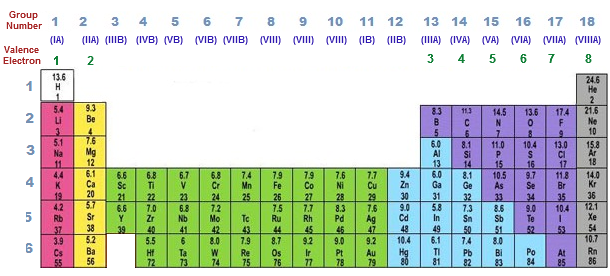# What is Valency With Examples & How Do We Calculate

The valency of an element is the potential or the tendency of an element to combine with the other atoms. So today we shall discuss on the topic What is Valency With Examples. It is especially the measure of how many Hydrogen atoms can an element can displace or combine with. All the elements in the same group in the periodic table have the same valency.

Given below is a table where valency of a few common elements is shown :### How Valency is Calculated For an Element

The valency of an element or number of valence electrons in an element can be determined by two methods.

The first method is by using a Periodic Table. You just need to look at the group (or column number) where the element belongs. It can determine the valency of an element.

For example, the element Magnesium is located in group 2, and hence has a valency of 2.### How Many Types of Valency Are There

There are two types of valencies. The first one is electrovalency and the second one is covalency.

Electrovalent _ If an element forms a bond with another element by losing or gaining an electron, it is called Electrovalency.

For example – Sodium Chloride (NaCl) is formed by the combining of sodium ion (Na) which is +1 and chlorine ion, which is -1.

Covalency – Covalency is the chemical reaction in which the atom of an element shares an electron with the atom of another element. The number of electrons needed to be shared to achieve the nearest inert gas electron configuration is known as its covalency.

So it shares its three electrons with the atom of another element to obtain an octet or the electronic configuration of an inert gas atom.

### How Do We Calculate Valency

It is very easy to determine the valency of an electron. There are two methods by which you can do so. You just have to look at the atomic number of the element in the Periodic Table. If the atomic number of the element is 4 or less, then the valency of the electron is equal to the number of electrons in the outer shell.

If the atomic number of an element is more than eight, then the valency is calculated by subtracting the number of electrons in the outer shell by 8.